|

# 恒洁新品亮相2018深圳室内设计年度颁奖盛典

专业设计看深圳。

6月26日，由深圳市室内设计师协会（SZAID）主办的2018深圳室内设计年度颁奖盛典隆重举行，两百余名深圳市室内设计师协会成员、以及深圳市各大设计机构代表共同见证诸多重量级设计奖项的颁发。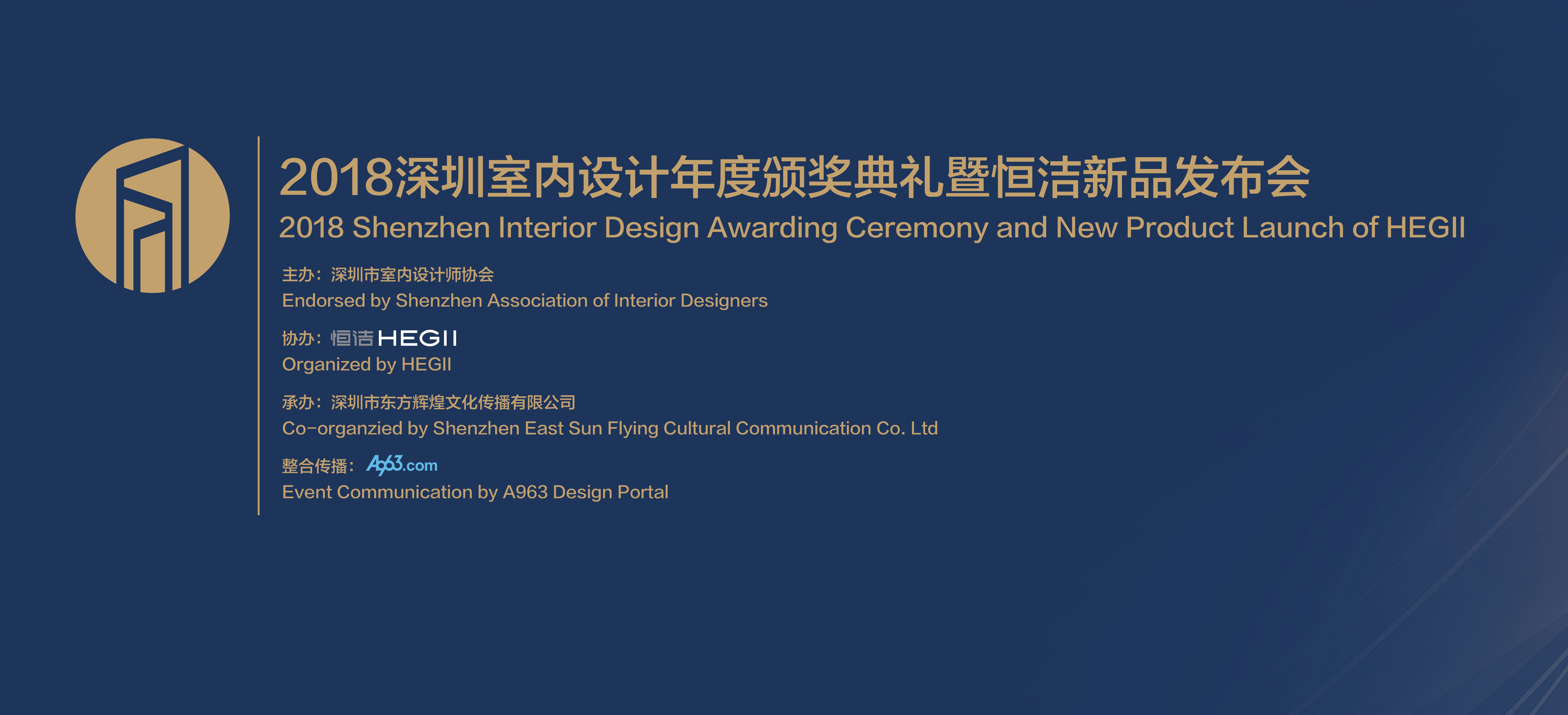同时，恒洁助力的《梦想改造家》特约设计师谢英凯也来到现场，与众多大咖一起探讨“设计点亮生活”。恒洁凭借着过硬的综合实力，在 2017年成为全国收视率第一的家装类节目——东方卫视《梦想改造家》唯一指定卫浴品牌后，2018年，恒洁将继续与《梦想改造家》节目展开深度合作。

另外，在这一设计大咖云集的颁奖典礼现场，恒洁简奢、摩登浴室柜新品以及Q10智能一体机重磅发布，以其美观、实用，科技感十足，而又绿色环保的特性，深受设计师群体的喜爱，成为现场瞩目的焦点。

专注出品，提升中国设计含金量

作为主办方的深圳室内设计师协会执行会长赵庆祥在开场致辞中指出，在新消费时代，只有注重设计与产业的联合发展，以设计驱动产业创新升级，才能引领消费升级，“而专注品质、持续创新的恒洁，以智能人性化的设计，致力于提升消费者的生活品质。”

活动特邀来宾、恒洁集团郑灵光总裁在现场发表热情致辞，并在致辞中提到，深圳作为经济特区飞速发展，而自加入联合国教科文组织全球创意城市网络后，成为了中国第一个、全球第六个“创意之都”，在全国设计界发挥着重要的引领作用。谈到恒洁，郑灵光总裁表示：“专注品质、持续创新、倡导环保，是恒洁一直坚持的品牌使命。”恒洁希望推出的每一款产品都能帮助消费者更享受浴室空间，从而提升消费者的生活品质，而注重环保也是恒洁持之以恒的努力方向，在水资源匮乏的当下，恒洁研发了3.5L节水坐便器。“我们是为造福下一代而进行了现在的工作。”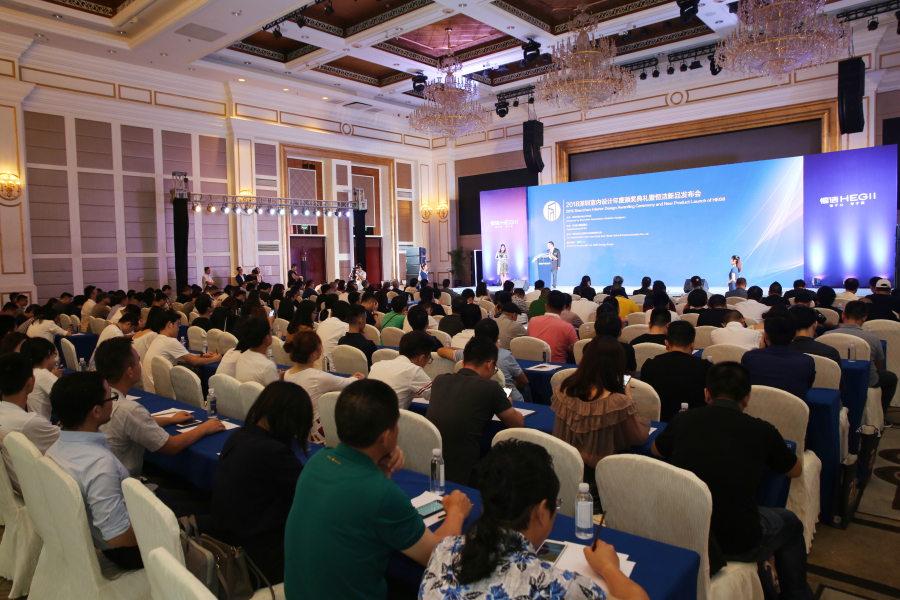绽放魅力，恒洁新品以设计圈粉

产品设计和室内空间设计密不可分，人性化的家居产品是优秀室内设计的重要组成部分。在本次颁奖盛典上另一个引人关注的焦点就是，恒洁简奢、摩登浴室柜新品以及Q10智能一体机在现场重磅发布，成为助力优秀室内设计的品质之选。

恒洁新品浴室柜的设计之道是颜值和实用的高度结合，简奢、摩登系列浴室柜，通过巧妙的收纳、对整体时尚感的把握、灵动的细节化处理以及五层防护等健康保障，为年轻人创造出自在、乐享、专属的卫浴空间。其中，简奢系浴室柜，整体优雅精炼、大气雅致、低调简洁，展现了一种新的生活方式；而摩登浴室柜，则通过个性的色彩元素、丰富艺术效果的材质、打破常规的操作方法，给人独一无二的专属体验。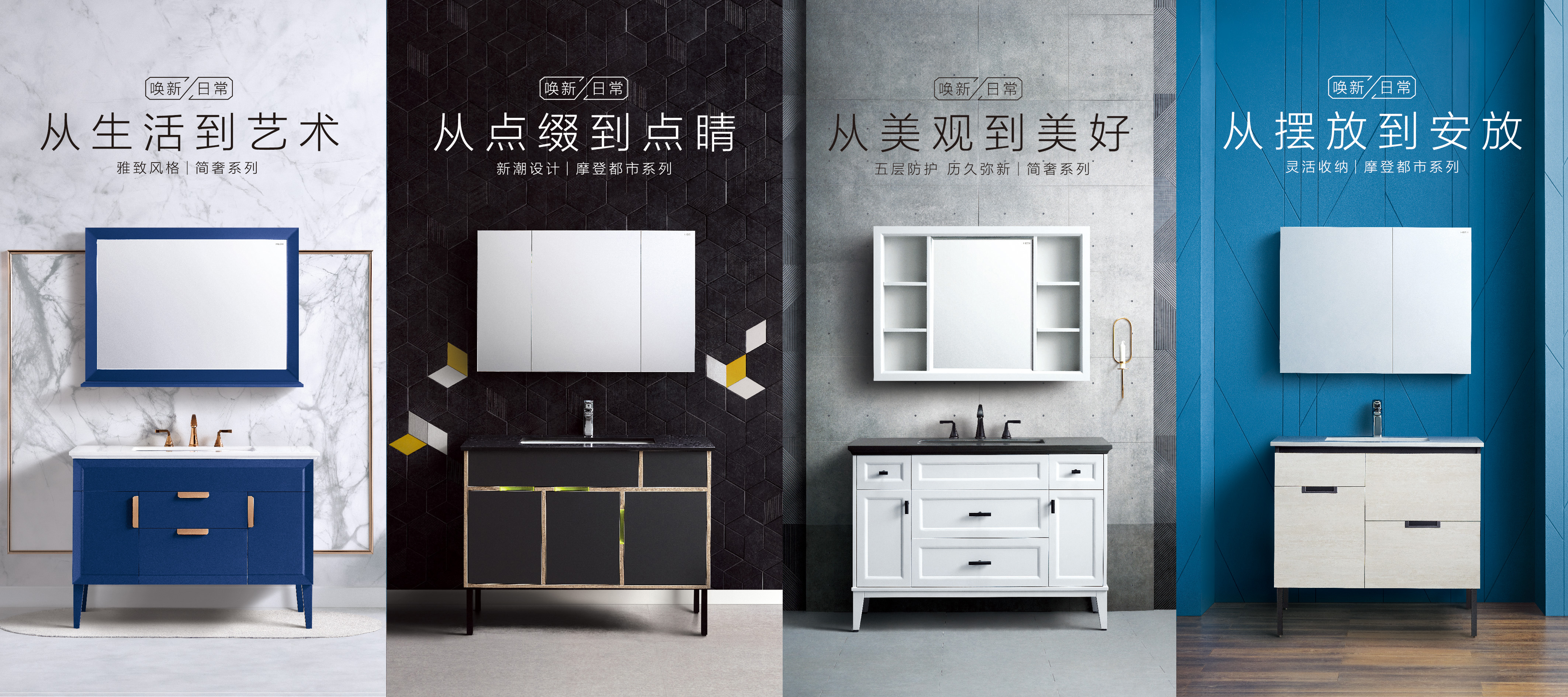Q10智能一体机也拥有诸多亮点，它除了搭载有活水即热、一键旋钮、水漩能冲水、智能翻盖四大指标性功能外，恒洁的工业设计师团队专门为Q10开发出智感即冲（男士小便自动冲水+离坐自动冲水）系统，杜绝了男士们因忘冲水而致的尴尬。由于新荷叶疏水材料的使用，Q10的盖板和座圈具有更高光洁度并能形成高硬度的疏水保护层，使表面更均匀、稳定。相比普通盖板和座圈，Q10在抗刮花、抗黄、抑菌效果上也更出色。这些智能化的设计，让参与活动的设计师们兴致勃勃。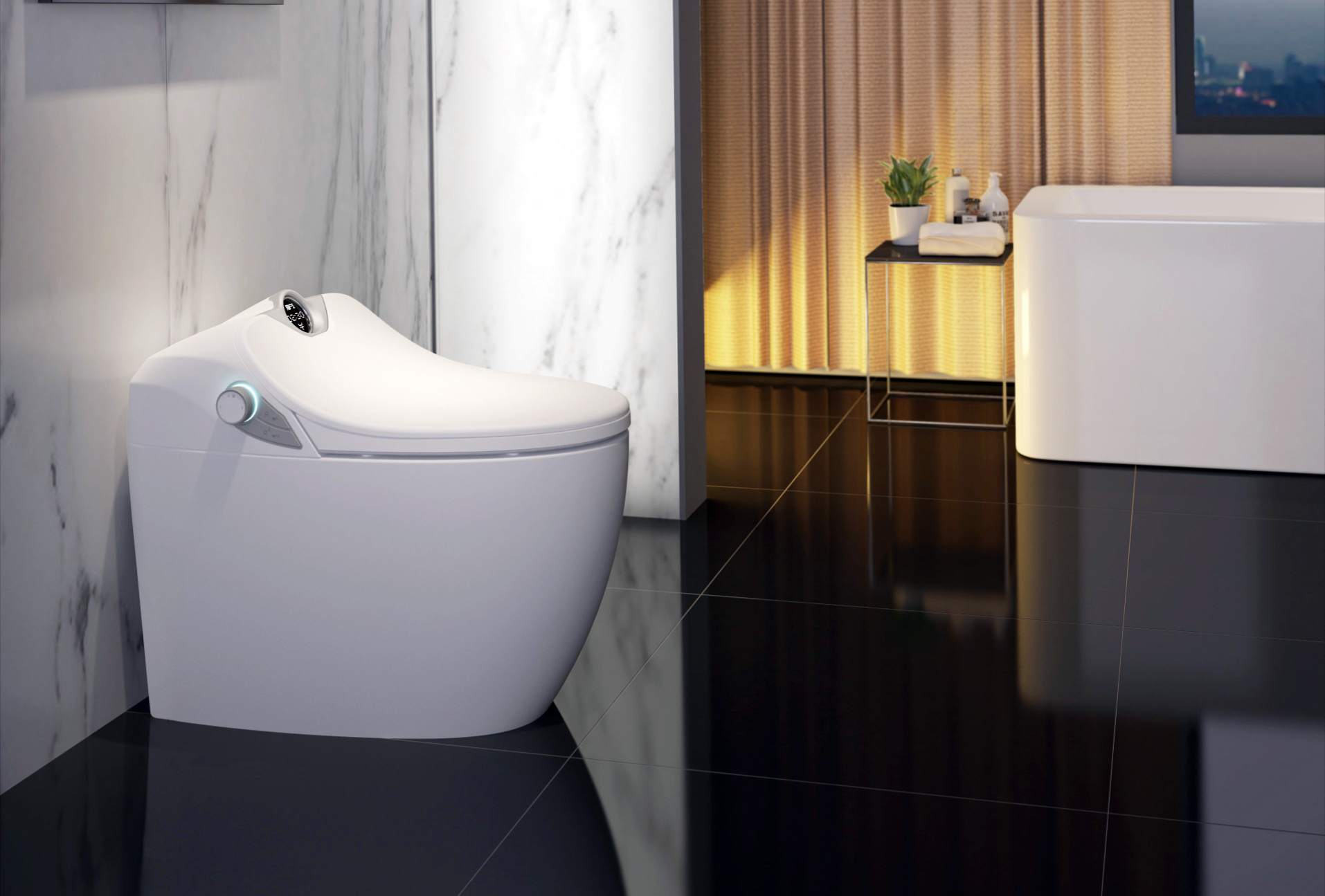恒洁的这些新品，以超高的产品品质和人性化的设计，在眼光独到的设计师群体中实力圈粉，新品发布环节引得现场拍照声不断。在活动间歇，大家更是在恒洁新品展示台前驻足流连，踊跃体验恒洁的人性化设计产品。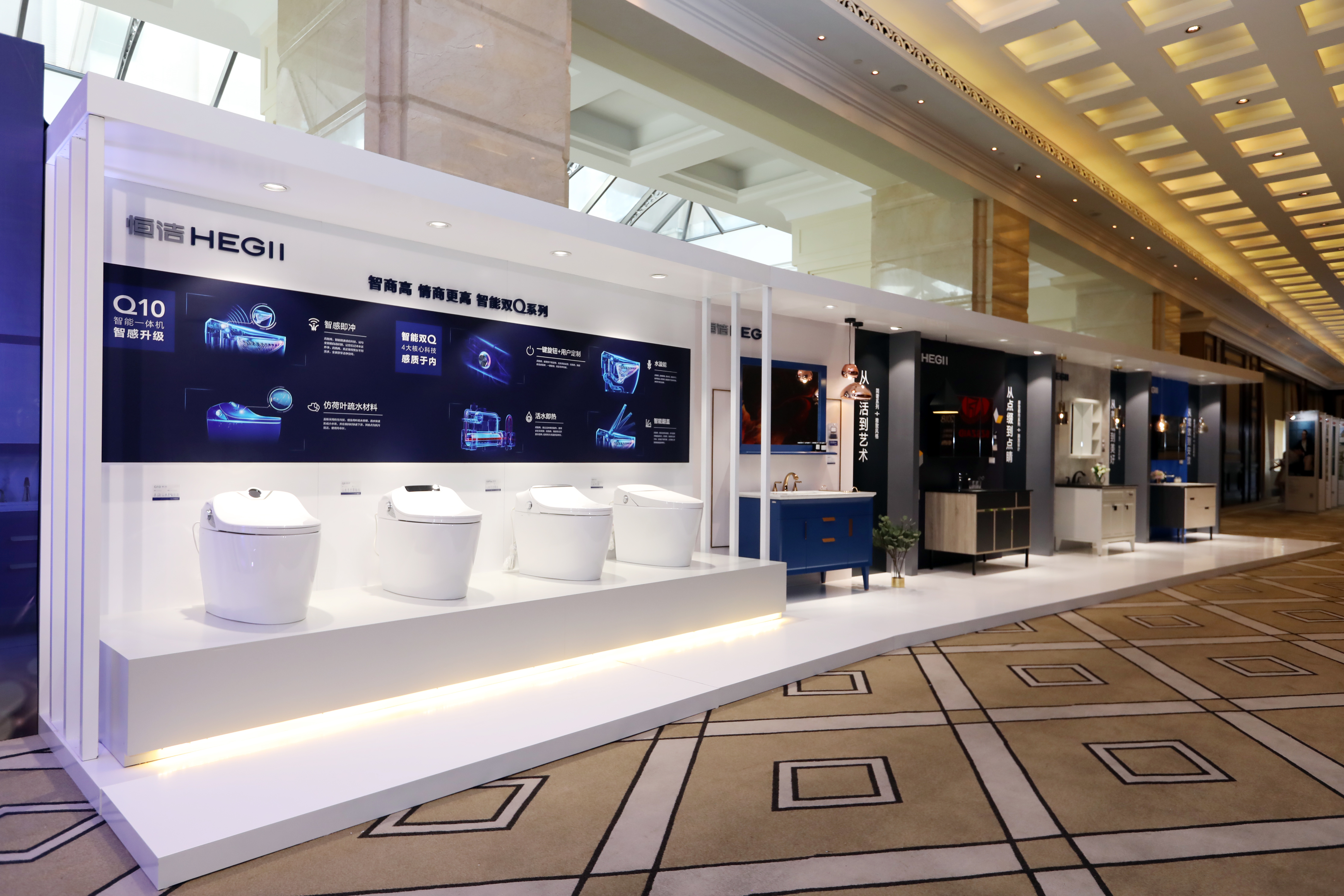大咖对话，解读未来设计趋势

2018深圳室内设计年度颁奖盛典现场，星光熠熠。包括IDEA-TOPS艾特奖获得者、深圳市室内设计师协会会长洪忠轩，恒洁《梦想改造家》特约设计师、汤物臣•肯文创意集团执行董事、设计总监谢英凯，恒洁卫浴工业设计总监姜弥弥等设计大咖都亲临现场，参与设计「智对话」，交流设计之道、探讨设计发展趋势。在「智对话」环节谈到恒洁产品设计方法论时，姜弥弥女士表示，用户是空间设计和工业设计的交汇点，恒洁产品设计都是基于消费者需求和使用习惯的洞察。恒洁与权威机构联合发布《2018中国卫浴消费趋势白皮书》正是体现了这一设计准则。在恒洁工业设计师团队看来，产品既是独立亮点，也要为整体空间传递出独特感染力；而「实用」永远是产品设计中的刚需，恒洁智能双Q就美观且实用，能帮助消费者解决使用痛点、创造出舒适体验，因此受到消费者的欢迎。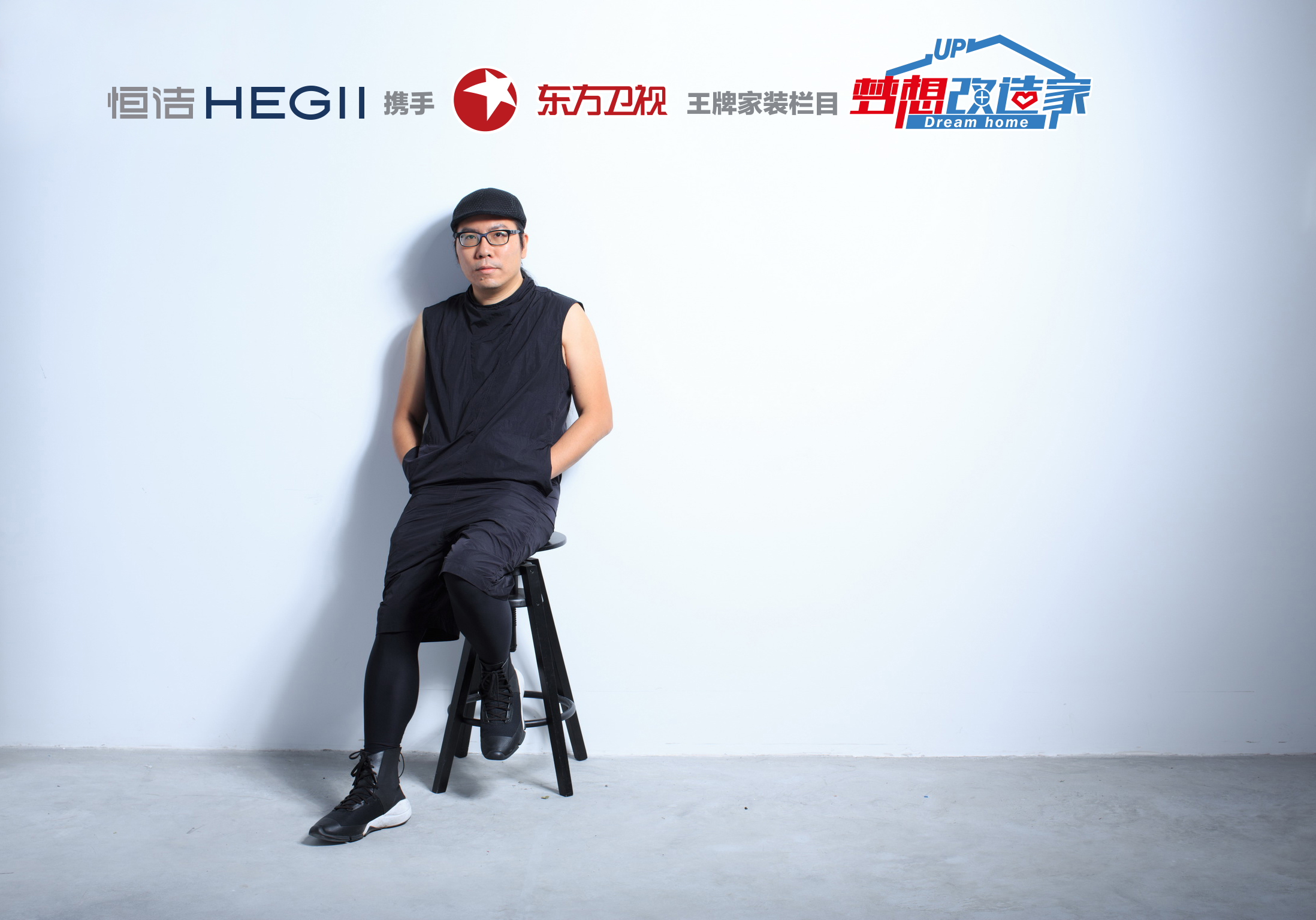知名设计师谢英凯因《梦想改造家》而与恒洁卫浴结缘，并通过恒洁产品圆满实现了在广州历史文化街区的百年老宅改造，因而对恒洁产品品质和恒洁产品中的设计用心有更深刻的感受。谈到智能化设计，谢英凯认为，时下流行的智能化不应该停仅留于噱头，而应该更关注人的需求和体验，满足人们对生活细致的体验和品质的需求，就像Q10智能一体机，它的智感即冲功能就特别照顾到老人和小孩，提升了他们的使用体验。

随着恒洁与设计界日趋深入的协作，双方正在「让人民生活更美好」的共同目标下创造多赢。未来，恒洁将继续专注品质、持续创新、倡导环保，以设计为支点，通过打造高品质产品将设计之美带入千家万户，让设计点亮生活。`声明：本文由入驻焦点开放平台的作者撰写，除焦点官方账号外，观点仅代表作者本人，不代表焦点立场错误信息举报电话： 400-099-0099，邮箱：jubao@vip.sohu.com，或点此进行意见反馈，或点此进行举报投诉。`A B C D E F G H J K L M N P Q R S T W X Y Z
A - B - C - D - E
• A
• 鞍山
• 安庆
• 安阳
• 安顺
• 安康
• 澳门
• B
• 北京
• 保定
• 包头
• 巴彦淖尔
• 本溪
• 蚌埠
• 亳州
• 滨州
• 北海
• 百色
• 巴中
• 毕节
• 保山
• 宝鸡
• 白银
• 巴州
• C
• 承德
• 沧州
• 长治
• 赤峰
• 朝阳
• 长春
• 常州
• 滁州
• 池州
• 长沙
• 常德
• 郴州
• 潮州
• 崇左
• 重庆
• 成都
• 楚雄
• 昌都
• 慈溪
• 常熟
• D
• 大同
• 大连
• 丹东
• 大庆
• 东营
• 德州
• 东莞
• 德阳
• 达州
• 大理
• 德宏
• 定西
• 儋州
• 东平
• E
• 鄂尔多斯
• 鄂州
• 恩施
F - G - H - I - J
• F
• 抚顺
• 阜新
• 阜阳
• 福州
• 抚州
• 佛山
• 防城港
• G
• 赣州
• 广州
• 桂林
• 贵港
• 广元
• 广安
• 贵阳
• 固原
• H
• 邯郸
• 衡水
• 呼和浩特
• 呼伦贝尔
• 葫芦岛
• 哈尔滨
• 黑河
• 淮安
• 杭州
• 湖州
• 合肥
• 淮南
• 淮北
• 黄山
• 菏泽
• 鹤壁
• 黄石
• 黄冈
• 衡阳
• 怀化
• 惠州
• 河源
• 贺州
• 河池
• 海口
• 红河
• 汉中
• 海东
• I
• J
• 晋中
• 锦州
• 吉林
• 鸡西
• 佳木斯
• 嘉兴
• 金华
• 景德镇
• 九江
• 吉安
• 济南
• 济宁
• 焦作
• 荆门
• 荆州
• 江门
• 揭阳
• 金昌
• 酒泉
• 嘉峪关
K - L - M - N - P
• K
• 开封
• 昆明
• 昆山
• L
• 廊坊
• 临汾
• 辽阳
• 连云港
• 丽水
• 六安
• 龙岩
• 莱芜
• 临沂
• 聊城
• 洛阳
• 漯河
• 娄底
• 柳州
• 来宾
• 泸州
• 乐山
• 六盘水
• 丽江
• 临沧
• 拉萨
• 林芝
• 兰州
• 陇南
• M
• 牡丹江
• 马鞍山
• 茂名
• 梅州
• 绵阳
• 眉山
• N
• 南京
• 南通
• 宁波
• 南平
• 宁德
• 南昌
• 南阳
• 南宁
• 内江
• 南充
• P
• 盘锦
• 莆田
• 平顶山
• 濮阳
• 攀枝花
• 普洱
• 平凉
Q - R - S - T - W
• Q
• 秦皇岛
• 齐齐哈尔
• 衢州
• 泉州
• 青岛
• 清远
• 钦州
• 黔南
• 曲靖
• 庆阳
• R
• 日照
• 日喀则
• S
• 石家庄
• 沈阳
• 双鸭山
• 绥化
• 上海
• 苏州
• 宿迁
• 绍兴
• 宿州
• 三明
• 上饶
• 三门峡
• 商丘
• 十堰
• 随州
• 邵阳
• 韶关
• 深圳
• 汕头
• 汕尾
• 三亚
• 三沙
• 遂宁
• 山南
• 商洛
• 石嘴山
• T
• 天津
• 唐山
• 太原
• 通辽
• 铁岭
• 泰州
• 台州
• 铜陵
• 泰安
• 铜仁
• 铜川
• 天水
• 天门
• W
• 乌海
• 乌兰察布
• 无锡
• 温州
• 芜湖
• 潍坊
• 威海
• 武汉
• 梧州
• 渭南
• 武威
• 吴忠
• 乌鲁木齐
X - Y - Z
• X
• 邢台
• 徐州
• 宣城
• 厦门
• 新乡
• 许昌
• 信阳
• 襄阳
• 孝感
• 咸宁
• 湘潭
• 湘西
• 西双版纳
• 西安
• 咸阳
• 西宁
• 仙桃
• 西昌
• Y
• 运城
• 营口
• 盐城
• 扬州
• 鹰潭
• 宜春
• 烟台
• 宜昌
• 岳阳
• 益阳
• 永州
• 阳江
• 云浮
• 玉林
• 宜宾
• 雅安
• 玉溪
• 延安
• 榆林
• 银川
• Z
• 张家口
• 镇江
• 舟山
• 漳州
• 淄博
• 枣庄
• 郑州
• 周口
• 驻马店
• 株洲
• 张家界
• 珠海
• 湛江
• 肇庆
• 中山
• 自贡
• 资阳
• 遵义
• 昭通
• 张掖
• 中卫

1室1厅1厨1卫1阳台

1
2
3
4
5

0
1
2

1

1

0
1
2
3报名成功，资料已提交审核A B C D E F G H J K L M N P Q R S T W X Y Z
A - B - C - D - E
• A
• 鞍山
• 安庆
• 安阳
• 安顺
• 安康
• 澳门
• B
• 北京
• 保定
• 包头
• 巴彦淖尔
• 本溪
• 蚌埠
• 亳州
• 滨州
• 北海
• 百色
• 巴中
• 毕节
• 保山
• 宝鸡
• 白银
• 巴州
• C
• 承德
• 沧州
• 长治
• 赤峰
• 朝阳
• 长春
• 常州
• 滁州
• 池州
• 长沙
• 常德
• 郴州
• 潮州
• 崇左
• 重庆
• 成都
• 楚雄
• 昌都
• 慈溪
• 常熟
• D
• 大同
• 大连
• 丹东
• 大庆
• 东营
• 德州
• 东莞
• 德阳
• 达州
• 大理
• 德宏
• 定西
• 儋州
• 东平
• E
• 鄂尔多斯
• 鄂州
• 恩施
F - G - H - I - J
• F
• 抚顺
• 阜新
• 阜阳
• 福州
• 抚州
• 佛山
• 防城港
• G
• 赣州
• 广州
• 桂林
• 贵港
• 广元
• 广安
• 贵阳
• 固原
• H
• 邯郸
• 衡水
• 呼和浩特
• 呼伦贝尔
• 葫芦岛
• 哈尔滨
• 黑河
• 淮安
• 杭州
• 湖州
• 合肥
• 淮南
• 淮北
• 黄山
• 菏泽
• 鹤壁
• 黄石
• 黄冈
• 衡阳
• 怀化
• 惠州
• 河源
• 贺州
• 河池
• 海口
• 红河
• 汉中
• 海东
• I
• J
• 晋中
• 锦州
• 吉林
• 鸡西
• 佳木斯
• 嘉兴
• 金华
• 景德镇
• 九江
• 吉安
• 济南
• 济宁
• 焦作
• 荆门
• 荆州
• 江门
• 揭阳
• 金昌
• 酒泉
• 嘉峪关
K - L - M - N - P
• K
• 开封
• 昆明
• 昆山
• L
• 廊坊
• 临汾
• 辽阳
• 连云港
• 丽水
• 六安
• 龙岩
• 莱芜
• 临沂
• 聊城
• 洛阳
• 漯河
• 娄底
• 柳州
• 来宾
• 泸州
• 乐山
• 六盘水
• 丽江
• 临沧
• 拉萨
• 林芝
• 兰州
• 陇南
• M
• 牡丹江
• 马鞍山
• 茂名
• 梅州
• 绵阳
• 眉山
• N
• 南京
• 南通
• 宁波
• 南平
• 宁德
• 南昌
• 南阳
• 南宁
• 内江
• 南充
• P
• 盘锦
• 莆田
• 平顶山
• 濮阳
• 攀枝花
• 普洱
• 平凉
Q - R - S - T - W
• Q
• 秦皇岛
• 齐齐哈尔
• 衢州
• 泉州
• 青岛
• 清远
• 钦州
• 黔南
• 曲靖
• 庆阳
• R
• 日照
• 日喀则
• S
• 石家庄
• 沈阳
• 双鸭山
• 绥化
• 上海
• 苏州
• 宿迁
• 绍兴
• 宿州
• 三明
• 上饶
• 三门峡
• 商丘
• 十堰
• 随州
• 邵阳
• 韶关
• 深圳
• 汕头
• 汕尾
• 三亚
• 三沙
• 遂宁
• 山南
• 商洛
• 石嘴山
• T
• 天津
• 唐山
• 太原
• 通辽
• 铁岭
• 泰州
• 台州
• 铜陵
• 泰安
• 铜仁
• 铜川
• 天水
• 天门
• W
• 乌海
• 乌兰察布
• 无锡
• 温州
• 芜湖
• 潍坊
• 威海
• 武汉
• 梧州
• 渭南
• 武威
• 吴忠
• 乌鲁木齐
X - Y - Z
• X
• 邢台
• 徐州
• 宣城
• 厦门
• 新乡
• 许昌
• 信阳
• 襄阳
• 孝感
• 咸宁
• 湘潭
• 湘西
• 西双版纳
• 西安
• 咸阳
• 西宁
• 仙桃
• 西昌
• Y
• 运城
• 营口
• 盐城
• 扬州
• 鹰潭
• 宜春
• 烟台
• 宜昌
• 岳阳
• 益阳
• 永州
• 阳江
• 云浮
• 玉林
• 宜宾
• 雅安
• 玉溪
• 延安
• 榆林
• 银川
• Z
• 张家口
• 镇江
• 舟山
• 漳州
• 淄博
• 枣庄
• 郑州
• 周口
• 驻马店
• 株洲
• 张家界
• 珠海
• 湛江
• 肇庆
• 中山
• 自贡
• 资阳
• 遵义
• 昭通
• 张掖
• 中卫• 手机• 分享
• 设计
免费设计
• 计算器
装修计算器
• 入驻
合作入驻
• 联系
联系我们
• 置顶
返回顶部